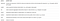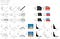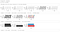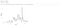# Automated Graphical Exploratory Data Analysis with brinton

The `brinton` package has been created to facilitate exploratory data analysis following the visual information-seeking mantra: “Overview first, zoom and filter, then details on demand”. The main idea is to assist the user during these three phases through three functions: `wideplot()`, `longplot()` and `plotup()`. While each of these functions has its own arguments and purpose, all three serve to facilitate exploratory data analysis and the selection of a suitable graphic.

The package can be installed easily from the Comprehensive R Archive Network (CRAN) using the R console. When the package is loaded into memory, it provides a startup message that pays homage to Henry D. Hubbard’s enthusiastic introduction to the book Graphic Presentation by Willard Cope Brinton in 1939:

`> install.packages('brinton')> library(brinton)M a G i C i N G R a P H S`

The package includes up to now only 3 functions. The `wideplot()` function allows the user to explore a dataset as a whole using a grid of graphics in which each variable is represented through multiple graphics. Once we have explored the dataset as a whole, the `longplot()` allows us to explore other graphics for a given variable. This function also presents a grid of graphics, but instead of showing a selection of graphics for each variable, it presents the full array of graphics available in the library to represent a single variable. Once we have narrowed in on a certain graphic, we can use the `plotup()` function, which presents the values of a variable on a single graphic. We can access the code of the resulting graph and adapt it as needed. These three functions expand the graphic types that are presented automatically by the autoGEDA libraries in the R environment.

# The wideplot function

The `wideplot()` function returns a graphical summary of the variables included in the dataset to which it has been applied. First it groups the variables according to the following sequence: `logical`, `ordered`, `factor`, `character`, `datetime`, `numeric`. Next, it creates a multipanel graphic in html format, in which each variable of the dataset is represented in a row of the grid, while each column displays the different graphics possible for each variable. We called the resulting graphic type wideplot because it shows an array of graphics for all of the columns of the dataset. The structure of the function, the arguments it permits and its default values are as follows:

`wideplot(data, dataclass = NULL, logical = NULL, ordered = NULL,  factor = NULL, character = NULL, datetime = NULL, numeric = NULL,  group = NULL, ncol = 7, label = 'FALSE')`

The only argument necessary to obtain a result is `data` that expects a `data-frame` class object; `ncol` filters the first n columns of the grid, between 3 and 7, which will be shown. The fewer columns displayed, the larger the size of the resulting graphics, a feature that is especially useful if the scale labels dwarf the graphics area; `label` adds to the grid a vector below each group of rows according to the variable type, with the names and order of the graphics; `logical`, `ordered`, `factor`, `character`, `datetime` and `numeric` make it possible to choose which graphics appear in the grid and in what order, for each variable type. Finally, `group` changes the selection of graphics that are shown by default according to the criteria of the following table.Available values for the ‘group’ argument

The `wideplot()` function takes inspiration from this function, but instead of describing the dataset in textual or tabular form, it does it graphically. We can easily compare the results of these two functions, for example, with the dataset esoph from a case-control study of esophageal cancer in Ille-et-Vilaine, France. The dataset has three ordered factor-type variables and two numerical variables:

`str(esoph)## 'data.frame':    88 obs. of  5 variables:##  \$ agegp    : Ord.factor w/ 6 levels "25-34"<"35-44"<..: 1 1 1 1 ##  1 1 1 1 1 1 ...##  \$ alcgp    : Ord.factor w/ 4 levels "0-39g/day"<"40-79"<..: 1 1 ##  1 1 2 2 2 2 3 3 ...##  \$ tobgp    : Ord.factor w/ 4 levels "0-9g/day"<"10-19"<..: 1 2 3 ##  4 1 2 3 4 1 2 ...##  \$ ncases   : num  0 0 0 0 0 0 0 0 0 0 ...##  \$ ncontrols: num  40 10 6 5 27 7 4 7 2 1 ...wideplot(esoph)`Output of wideplot(esoph)

If the order and graphic types to be shown for each variable type are not specified and if the graphic types aren’t filtered using the argument `group`, then the default graphic will contain an opinion-based selection graphics for each variable type, organized especially to facilitate comparison between graphics of the same row and between graphics of the same column. The user can overwrite this default selection of graphics as needed, using the arguments `logical`, `ordered`, `factor`, `character`, `datetime` and `numeric`.

# The longplot function

To facilitate economy of calculation, the `wideplot()` function presents a limited number of graphics in each row. If the user wants to expand the array of suggested graphics for a given variable, he or she should use the `longplot()` function, which returns a grid with all of the graphics considered by the library for that variable. The structure of the function is very simple `longplot(data, vars, label = TRUE)` and we can easily check the outcome of applying this function to the variable `alcgp` of the dataset `esoph`:

`longplot(esoph, 'alcgp')`Output of longplot(esoph, 'alcgp')

The arguments of the function are `data`, which must be a `data-frame` class object; `vars`, which requires the name of a specific variable of the dataset; and `label`, which does not have to be defined and which adds a vector below each row of the grid indicating the name of each graphic. Unlike the grid of the `wideplot()` function, the grid of the `longplot()` function does not include parameters to limit the array of graphics to be presented. We made this decision because the main advantage of this function is precisely that it presents all of the graphic representations available for a given variable. However, we do not rule out adding filters that limit the number of graphics to be shown if this feature seems useful as the catalog fills with graphics. Each graphic presented can be called explicitly by name using the functions `wideplot()` and `plotup()`, which is why the argument `label` has been set to `TRUE` in this case.

The array of graphics that the `longplot()` function returns is sorted so that in the rows we find different graphic types and in the columns different variations of the same graphic type. This organization, however, is not absolute and in some cases in order to compress the results, we find different graphic types in the columns of the same row.

# The plotup function

The `plotup()` function has the following structure: `plotup(data, vars, diagram, output = 'html')`. By default, this function returns an html document with a single graphic based on a variable from a given dataset and the name of the desired graphic, from among the names included by the specimen that we present in the next subsection. We can easily check the outcome of applying this function to produce a line graph from the variable `ncases` of the dataset `esoph`:

`plotup(esoph, 'ncases', 'line graph')`Output of plotup(esoph, 'ncases', 'line graph')

This function requires three arguments: `data`, `vars` and `diagram`. The fourth argument, `output`, is optional and has the default value of html. However, if it is set to `plots pane`, instead of generating a graphic in an html page, it generates a graphic in the plots pane of RStudio. If, instead, it is set to ’’console´´, the function returns the code used by the library to generate this precise graphic. This feature is especially useful to adapt the default graphic to the specific needs and preferences of the user.

`plotup(data = esoph, vars = 'ncases', diagram ='line graph', output = 'console')`

This returns the following code:

`theme_set(theme_minimal())ggplot(esoph, aes(x=seq_along(ncases), y=ncases)) +  geom_line() +  labs(x='seq') +  theme(panel.grid = element_line(colour = NA),    axis.ticks = element_line(color = 'black'))`

# The specimen

The documentation of the library includes the vignette ‘1v specimen’, which contains a specimen with images of all the graphic types for a single variable, incorporated into the library according to the variable type. These graphs serve as an example so that the user can rapidly check whether a graphic has been incorporated, the type or types of variable for which it has been incorporated, and the label with which it has been identified. The suitability of a particular graphic will depend on the datasets of interest and the variables of each particular user.

In order to keep the package as compact as possible, the specimens of graphics that require more than one input variable are not built whithin the package but they can be found at sciencegraph.github.io/brinton/articles/.

This article is a short version of the paper originally published at https://journal.r-project.org/archive/2021/RJ-2021-018/index.html coauthored by Ramon Oller Piqué. If you wish to cite the original source you may write:

Millán-Martínez P, Oller R (2021). “A Graphical EDA Tool with ggplot2: brinton.” The R Journal, *12*(2), 631. doi: 10.32614/RJ-2021–018 (URL: https://journal.r-project.org/archive/2021/RJ-2021-018/index.html)